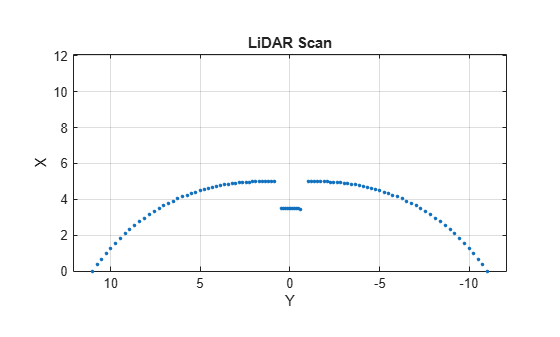Main Content

# plot

Display laser or lidar scan readings

## Syntax

``plot(scanObj)``
``plot(___,Name,Value)``
``linehandle = plot(___)``

## Description

example

````plot(scanObj)` plots the lidar scan readings specified in `scanObj`.```
````plot(___,Name,Value)` provides additional options specified by one or more `Name,Value` pair arguments.```
````linehandle = plot(___)` returns a column vector of line series handles, using any of the arguments from previous syntaxes. Use `linehandle` to modify properties of the line series after it is created.```

## Examples

collapse all

Specify lidar data as vectors of ranges and angles. These values include readings outside of the sensors range.

```x = linspace(-2,2); ranges = abs((1.5).*x.^2 + 5); ranges(45:55) = 3.5; angles = linspace(-pi/2,pi/2,numel(ranges));```

Create a lidar scan by specifying the ranges and angles. Plot all points of the lidar scan.

```scan = lidarScan(ranges,angles); plot(scan)```Remove invalid points based on a specified minimum and maximum range.

```minRange = 0.1; maxRange = 7; scan2 = removeInvalidData(scan,'RangeLimits',[minRange maxRange]); hold on plot(scan2) legend('All Points','Valid Points')```## Input Arguments

collapse all

Lidar scan readings, specified as a `lidarScan` object.

### Name-Value Pair Arguments

Specify optional comma-separated pairs of `Name,Value` arguments. `Name` is the argument name and `Value` is the corresponding value. `Name` must appear inside quotes. You can specify several name and value pair arguments in any order as `Name1,Value1,...,NameN,ValueN`.

Example: `"MaximumRange",5`

Parent of axes, specified as the comma-separated pair consisting of `"Parent"` and an axes object in which the laser scan is drawn. By default, the laser scan is plotted in the currently active axes.

Range of laser scan, specified as the comma-separated pair consisting of `"MaximumRange"` and a scalar. When you specify this name-value pair argument, the minimum and maximum x-axis and the maximum y-axis limits are set based on specified value. The minimum y-axis limit is automatically determined by the opening angle of the laser scanner.

This name-value pair only works when you input `scanMsg` as the laser scan.

## Outputs

collapse all

One or more chart line objects, returned as a scalar or a vector. These are unique identifiers, which you can use to query and modify properties of a specific chart line.

## See Also

Introduced in R2015a